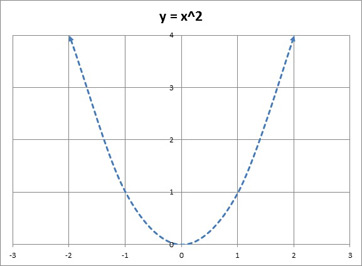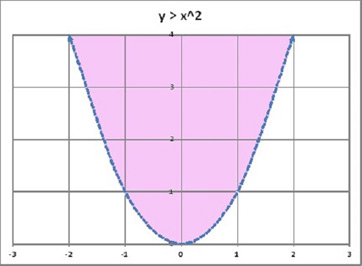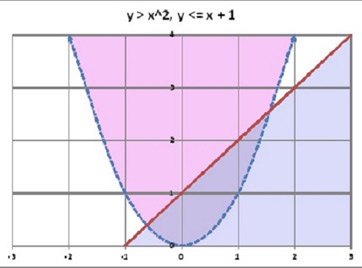# System of Inequalities: Graphing & Concept

An error occurred trying to load this video.

Try refreshing the page, or contact customer support.

Coming up next: What is a Matrix?

### You're on a roll. Keep up the good work!

Replay
Your next lesson will play in 10 seconds
• 0:04 Solving A System of…
• 0:27 Graphing A Single Inequality
• 1:58 The Second Inequality
• 2:50 The Final Solution
• 3:57 Lesson Summary

Want to watch this again later?

Timeline
Autoplay
Autoplay
Speed

#### Recommended Courses for You

Lesson Transcript
Instructor: Glenda Boozer
When we need to solve a system of two or more inequalities, we graph the solution set of each one, but we put them on the same graph and see what that looks like. The overlapping shaded areas tells us where to find the solution set.

## Solving a System of Inequalities

When we have a system of two or more inequalities to solve, we will graph the solution set of each one, but we will put them on the same graph and see what that looks like. The overlapping shaded areas will tell us where to find the solution set. For example, let's try this system:

y > x^2

y <= x + 1

## Graphing a Single Inequality

We will start by choosing one of the inequalities and graphing the equation associated with it. An equation is a statement that two quantities are equal. We can create that equation by replacing the >, <, >=, or <= signs by an = sign. For example, if we have y > x^2, we'll graph the equation y = x^2:We used a dotted line here instead of a solid line because points along the line are not included in the solution set, or the set of all solutions to the equation. How do we know? Let's take the point (2,4). In our inequality we replace x with 2 and y with 4, and we have 4 > 2^2; but 4 is not greater than 2^2, it is equal. Therefore, this point is not part of the solution set.

We have only made the boundary of the solution set so far; we need the graph to show all of the points where y is greater than x^2. Let's choose a point on the graph and see if it is part of the solution set. We'll try the point (0,1) since it is definitely above the dotted line. In fact, if it is part of the solution set, everything above the dotted line will be part of the solution set. Once more, we substitute 0 (the first number) for x, and the 1 (the second number) for y in the inequality, and we have 1 > 0^2. Hey, that's true! We can shade the area above the line:## The Second Inequality

Next, we graph the line y = x + 1 on the same grid. We will use a solid line here, because the inequality is y <= x + 1 and not just y < x + 1. You can see it in the graph as a straight, solid red line. Which side of the line should we shade? Let's test the point (0,0) and see if its x and y values will make the inequality true. We get 0 <= 0 + 1; this is true, so we shade the side that includes the point (0,0). This graph uses pale blue shading for the solution set of y <= x +1 to make it easier to see the solution sets of both inequalities.If we had three ineualities, or four, or any number, we would graph them on the same grid, using the same method.

To unlock this lesson you must be a Study.com Member.

### Register to view this lesson

Are you a student or a teacher?

### Unlock Your Education

#### See for yourself why 30 million people use Study.com

##### Become a Study.com member and start learning now.
Back
What teachers are saying about Study.com

### Earning College Credit

Did you know… We have over 160 college courses that prepare you to earn credit by exam that is accepted by over 1,500 colleges and universities. You can test out of the first two years of college and save thousands off your degree. Anyone can earn credit-by-exam regardless of age or education level.